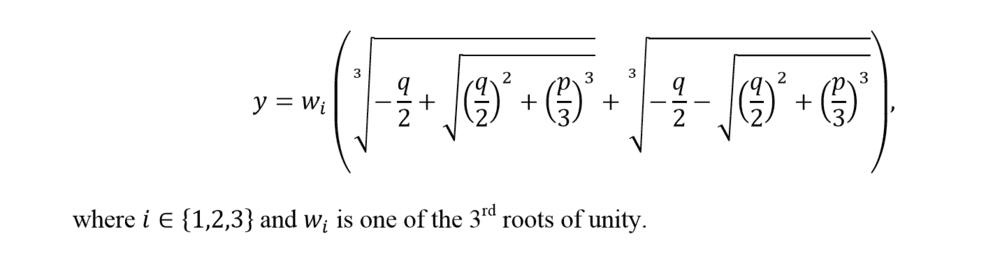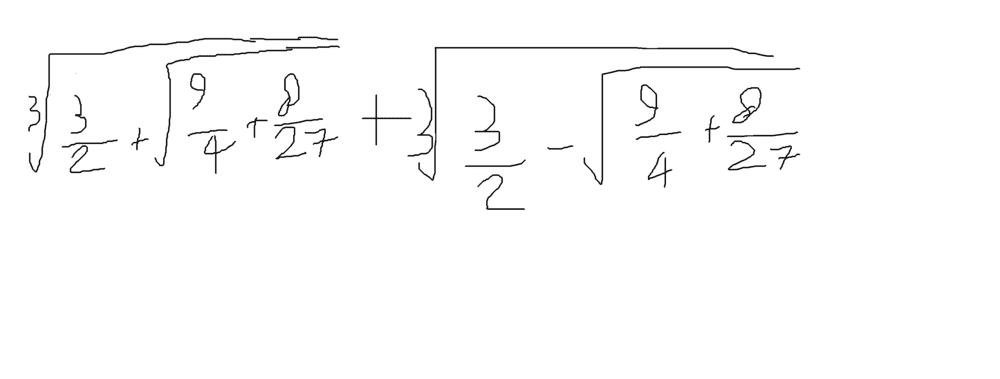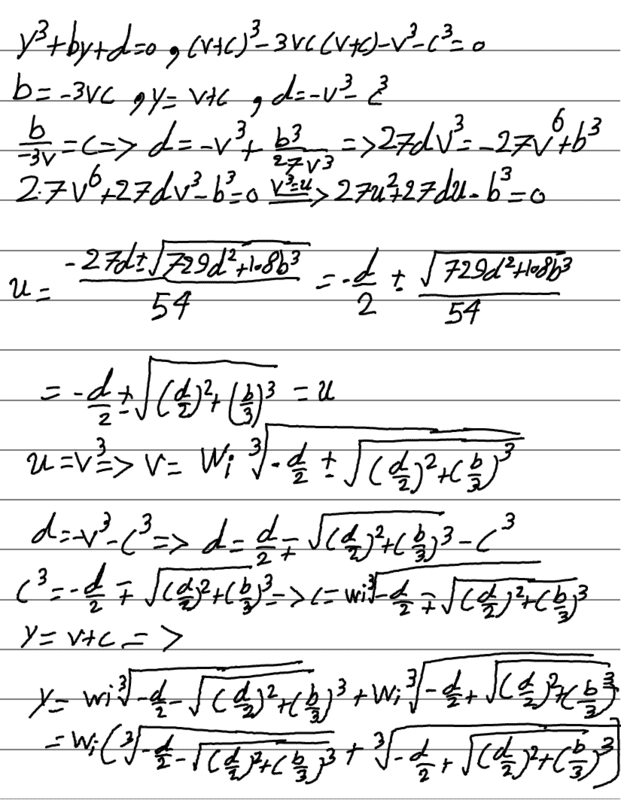# Cubic roots

• ali PMPAINT
ali PMPAINT
Homework Statement
Relevant Equations
PictureSo, you can prove it by using (v+c)^3-3vc(v+c)-(v^3+c^3)=0, where v+c=y and -3vc=p and v^3+c^3=q. The problem is, when I try to solve a one, I just get one answer(it should be 3) and I don't know what the article meant by wi. What am I missing?

Mentor
ali PMPAINT said:
, post: 6177224, member: 661503"]
Problem Statement: the answer to y^3+py+q=0
Relevant Equations: Picture

View attachment 243310
So, you can prove it by using (v+c)^3-3vc(v+c)-(v^3+c^3)=0, where v+c=y and -3vc=p and v^3+c^3=q.
I think you have a sign error. ##-(v^3 + c^3)## should be ##+(v^3 + c^3)## according to the substitution you show
ali PMPAINT said:
The problem is, when I try to solve a one, I just get one answer(it should be 3) and I don't know what the article meant by wi. What am I missing?
It says that ##w_i## is one of the cube roots of unity (1). In polar form these are
##w_0= 1##
##w_1 = \cos(\frac {2\pi}3) + i\sin(\frac {2\pi}3)##
##w_2 = \cos(\frac {4\pi}3) + i\sin(\frac {4\pi}3)##
Notice that two of these roots are complex.
If you raise any of these to the 3rd power, you get 1.

•ali PMPAINT
Staff Emeritus
Homework Helper
Gold Member
So, you can prove it by using (v+c)^3-3vc(v+c)-(v^3+c^3)=0, where v+c=y and -3vc=p and v^3+c^3=q.
I think you have a sign error. −(v3+c3)−(v3+c3)-(v^3 + c^3) should be +(v3+c3)+(v3+c3)+(v^3 + c^3) according to the substitution you show.
... or that should say: ##\ -(v^3+c^3) = q \, . ##

Also, for the casual reader, this expression, given by OP, is an identity : ##\ (v+c)^3-3vc(v+c)-(v^3+c^3)=0\, . ##

•ali PMPAINT
ali PMPAINT
I think you have a sign error. ##-(v^3 + c^3)## should be ##+(v^3 + c^3)## according to the substitution you show
Yes, thanks for correcting me.

It says that ##w_i## is one of the cube roots of unity (1). In polar form these are
##w_0= 1##
##w_1 = \cos(\frac {2\pi}3) + i\sin(\frac {2\pi}3)##
##w_2 = \cos(\frac {4\pi}3) + i\sin(\frac {4\pi}3)##
Notice that two of these roots are complex.
If you raise any of these to the 3rd power, you get 1.
So, I am now familiar a lot with polar form, so if we got an answer like 1 for example, do you set it equal to e^(ix)=cos(x)+isin(x) ?

Mentor
Yes, thanks for correcting me.

So, I am now familiar a lot with polar form, so if we got an answer like 1 for example, do you set it equal to e^(ix)=cos(x)+isin(x) ?
Here x is 0, so ##e^{i0} = \cos(0) + i\sin(0) = 1 + i0 = 1##

ali PMPAINT
Here x is 0, so ##e^{i0} = \cos(0) + i\sin(0) = 1 + i0 = 1##
For example, how to get all solutions for x^3+2x-3=0 ?

Last edited:
Gold Member
For example, how to get all solutions for x^3+2x-3=0 ?
do you know what synthetic division is?

Mentor
For example, how to get all solutions for x^3+2x-3=0 ?
You included the formula in your first post of this thread. I gave you all three roots of unity in my previous post. Just use that formula, once for each of ##w_0, w_1, w_2##.

Staff Emeritus
Homework Helper
Gold Member
It says that ##w_i## is one of the cube roots of unity (1). In polar form these are
##w_0= 1##
##w_1 = \cos(\frac {2\pi}3) + i\sin(\frac {2\pi}3)##
##w_2 = \cos(\frac {4\pi}3) + i\sin(\frac {4\pi}3)##
Notice that two of these roots are complex.
If you raise any of these to the 3rd power, you get 1.

So, I am now familiar a lot with polar forms, so if we got an answer like 1 for example, do you set it equal to e^(ix)=cos(x)+isin(x) ?
Here x is 0, so ##e^{i0} = \cos(0) + i\sin(0) = 1 + i0 = 1##
You can get ##w_1## by letting x = 2π/3 in the polar form you gave, which was: e^(ix)=cos(x)+isin(x)

Then ##w_1 = \cos(\frac {2\pi}3) + i\sin(\frac {2\pi}3)## which is the same as ##\displaystyle e^{2\pi i / 3} ##

It then follows that ##\displaystyle {w_1}^3=\left( e^{2\pi i / 3} \right) ^3 = e^{2\pi i } =1 ##

•ali PMPAINT
ali PMPAINT
You can get ##w_1## by letting x = 2π/3 in the polar form you gave, which was: e^(ix)=cos(x)+isin(x)

Then ##w_1 = \cos(\frac {2\pi}3) + i\sin(\frac {2\pi}3)## which is the same as ##\displaystyle e^{2\pi i / 3} ##

It then follows that ##\displaystyle {w_1}^3=\left( e^{2\pi i / 3} \right) ^3 = e^{2\pi i } =1 ##
Thanks, but now I'm facing another problem. When I tried to solve for x^3+2x-3=0 I got:I calculated it with a calculator and I got 1 out of this mess(which is true). No matter what I do, I can't simplify the expresion to get 1. And, I found that the other two roots are number 2 (by both calculator and another methods), but I tried to multiply it with ##w_1 = \cos(\frac {2\pi}3) + i\sin(\frac {2\pi}3)## with one, but I got a wrong result (-1/2+i 3^(0.5)/2 ) which is wrong. Where am I making a mistake?
I am sorry if I am asking a lot, but I really want to know how to solve cubic equations by radicals.

Mentor
Thanks, but now I'm facing another problem. When I tried to solve for x^3+2x-3=0 I got:
View attachment 243371
I calculated it with a calculator and I got 1 out of this mess(which is true). No matter what I do, I can't simplify the expresion to get 1. And, I found that the other two roots are number 2 (by both calculator and another methods),
Yes, 1 is a solution of the equation ##x^3 + 2x - 3 = 0##, because if you replace x by 1, you get 0 = 0, which is a true statement.
However, 2 is not a solution of this equation, despite what your calculator and another method imply. The reason is that if you replace x in the equation by 2, you get ##2^3 + 2(2) - 3 = 9 \ne 0##.

Knowing that x = 1 is a solution, that means that (x - 1) is a factor of ##x^3 + 2x - 3##. If you divide the cubic polynomial by (x - 1), either by long division or by synthetic division that @StoneTemplePython mentioned, you get a quadratic polynomial that you can break down using the Quadratic Formula. The other two solutions of your equation are complex.
ali PMPAINT said:
but I tried to multiply it with ##w_1 = \cos(\frac {2\pi}3) + i\sin(\frac {2\pi}3)## with one, but I got a wrong result (-1/2+i 3^(0.5)/2 ) which is wrong. Where am I making a mistake?
I am sorry if I am asking a lot, but I really want to know how to solve cubic equations by radicals.

•ali PMPAINT
Staff Emeritus
Homework Helper
Gold Member
Thanks, but now I'm facing another problem. When I tried to solve for x^3+2x-3=0 I got:
View attachment 243371
I calculated it with a calculator and I got 1 out of this mess(which is true). No matter what I do, I can't simplify the expresion to get 1. And, I found that the other two roots are number 2 (by both calculator and another methods), but I tried to multiply it with ##w_1 = \cos(\frac {2\pi}3) + i\sin(\frac {2\pi}3)## with one, but I got a wrong result (-1/2+i 3^(0.5)/2 ) which is wrong. Where am I making a mistake?
I am sorry if I am asking a lot, but I really want to know how to solve cubic equations by radicals.
Yes. the above expression gives you 1 (unity).

It looks like the given expression is incorrect.
The correct results for the two complex solutions to ##x^3+2x-3=0## are ## \displaystyle x= \frac{-1 \pm i \sqrt{11}\,}{2} \,.##

A correct form for the solution to the cubic equation: ##x^3+px+q=0## is:

## \displaystyle w_k \left({\sqrt[{3}]{-{q \over 2}+{\sqrt {{q^{2} \over 4}+{p^{3} \over 27}}}}}+ w_k{\sqrt[{3}]{-{q \over 2}-{\sqrt {{q^{2} \over 4}+{p^{3} \over 27}}}} }\ \right) \ ,
\\ \displaystyle \text{where } w_k=e^{2k\pi i /3} \ .##

Note that for ##k \in \{1,\,2,\,3\,\},\ \ w_k ## is one of the three cube roots of unity.

The above may be expressed in a variety of forms.

•ali PMPAINT
ali PMPAINT
Oh yes, my bad, apparently I calculated the roots of x^3-3x+2=0 instead of x^3+2x-3=0 and missed the sign (don't blame me, they are too similar)
A correct form for the solution to the cubic equation: ##x^3+px+q=0## is:

## \displaystyle w_k \left({\sqrt[{3}]{-{q \over 2}+{\sqrt {{q^{2} \over 4}+{p^{3} \over 27}}}}}+ w_k{\sqrt[{3}]{-{q \over 2}-{\sqrt {{q^{2} \over 4}+{p^{3} \over 27}}}} }\ \right) \ ,
\\ \displaystyle \text{where } w_k=e^{2k\pi i /3} \ .##

So, I think that I had a mistake in my proof, and it doesn't give me a correct answer for more than two answers:Where is my mistake?